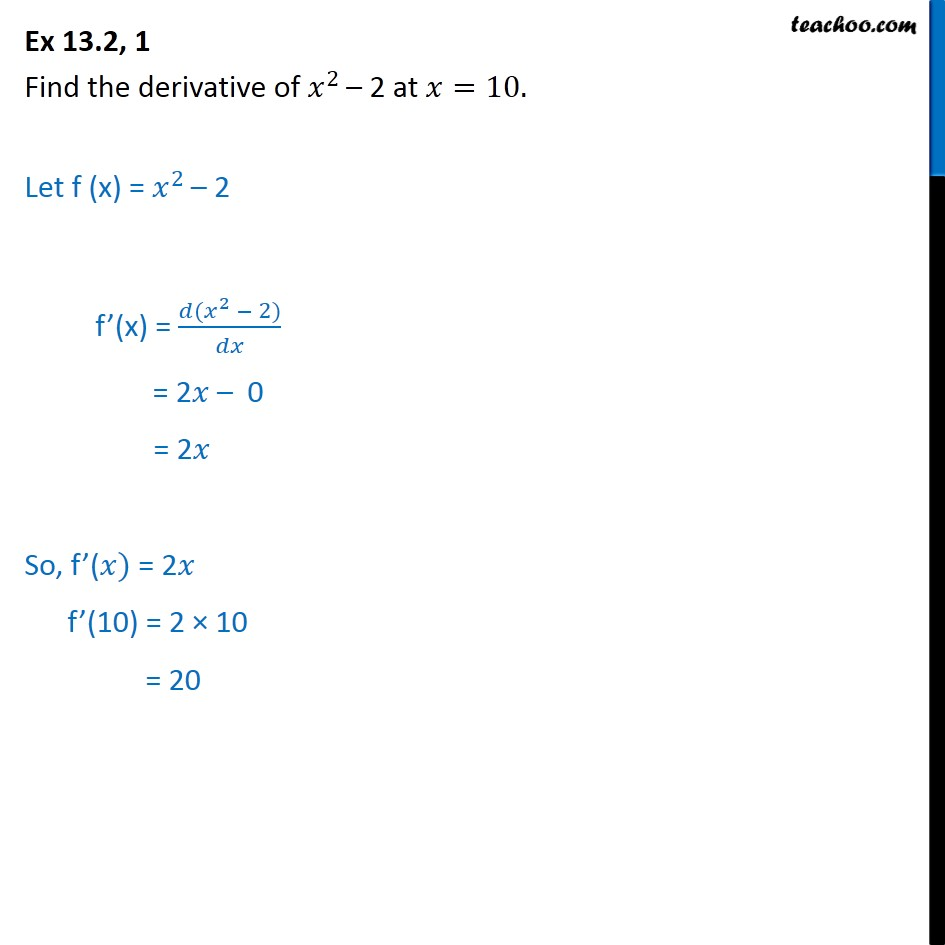1. Chapter 13 Class 11 Limits and Derivatives
2. Concept wise
3. Derivatives by 1st principle - At a point

Transcript

Ex 13.2, 1 Find the derivative of 𝑥﷮2 ﷯– 2 at 𝑥=10. Let f (x) = 𝑥﷮2 ﷯– 2 f’(x) = 𝑑( 𝑥﷮2﷯ − 2)﷮𝑑𝑥﷯ = 2𝑥 – 0 = 2𝑥 So, f’(𝑥) = 2𝑥 f’(10) = 2 × 10 = 20

Derivatives by 1st principle - At a point

About the AuthorDavneet Singh
Davneet Singh is a graduate from Indian Institute of Technology, Kanpur. He has been teaching from the past 10 years. He provides courses for Maths and Science at Teachoo.